### Home > CALC > Chapter 1 > Lesson 1.2.1 > Problem1-26

1-26.
1. Find the exact value of each of the following trig expressions. Homework Help ✎

1.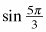2.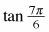3.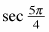4. csc π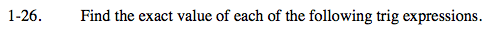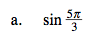Recall your unit circle, or the graph of f(x) = sinx.

$\text{Since reference point: sin}\frac{\pi}{3}=\frac{\sqrt{3}}{2},$

$\text{sin}\frac{5\pi}{3}\text{ will equal EITHER}\frac{\sqrt{3}}{2}\text{ or }-\frac{\sqrt{3}}{2}.$

Which one?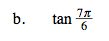$\text{tan}x=\frac{\text{sin}x}{\text{cos}x}$

$\text{tan}\frac{7\pi }{6}=\frac{\text{sin}\frac{7\pi }{6}}{\text{cos}\frac{7\pi }{6}}= \underline{ \ \ \ \ \ \ \ \ \ \ \ \ \ \ \ }$

Now simplify!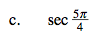$\text{sec}x=\frac{1}{\text{cos}x}$

$\text{sec}\frac{5\pi }{4}=\frac{1}{\text{cos}\frac{5\pi }{4}}= \underline{ \ \ \ \ \ \ \ \ \ \ \ \ \ \ \ }$

Now simplify!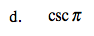$\text{csc}x=\frac{1}{\text{sin}x}$

Careful! sinπ = 0. What does that mean about the reciprocal of sinx?